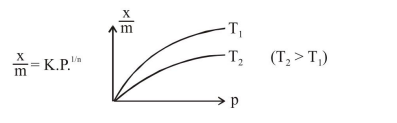# Adsorption of a gas follows Freundlich adsorption isotherm. If x is the mass of the gas

Question:

Adsorption of a gas follows Freundlich adsorption isotherm. If $x$ is the mass of the gas adsorbed on mass $\mathrm{m}$ of the adsorbent, the

correct plot of $\frac{x}{m}$ versus $p$ is :

1.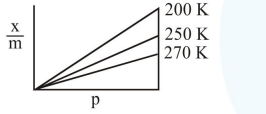2.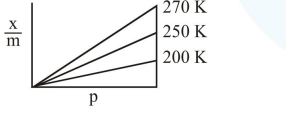3.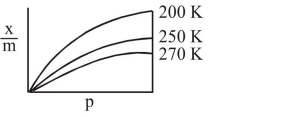4.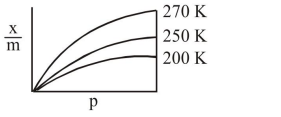Correct Option: , 3

Solution: